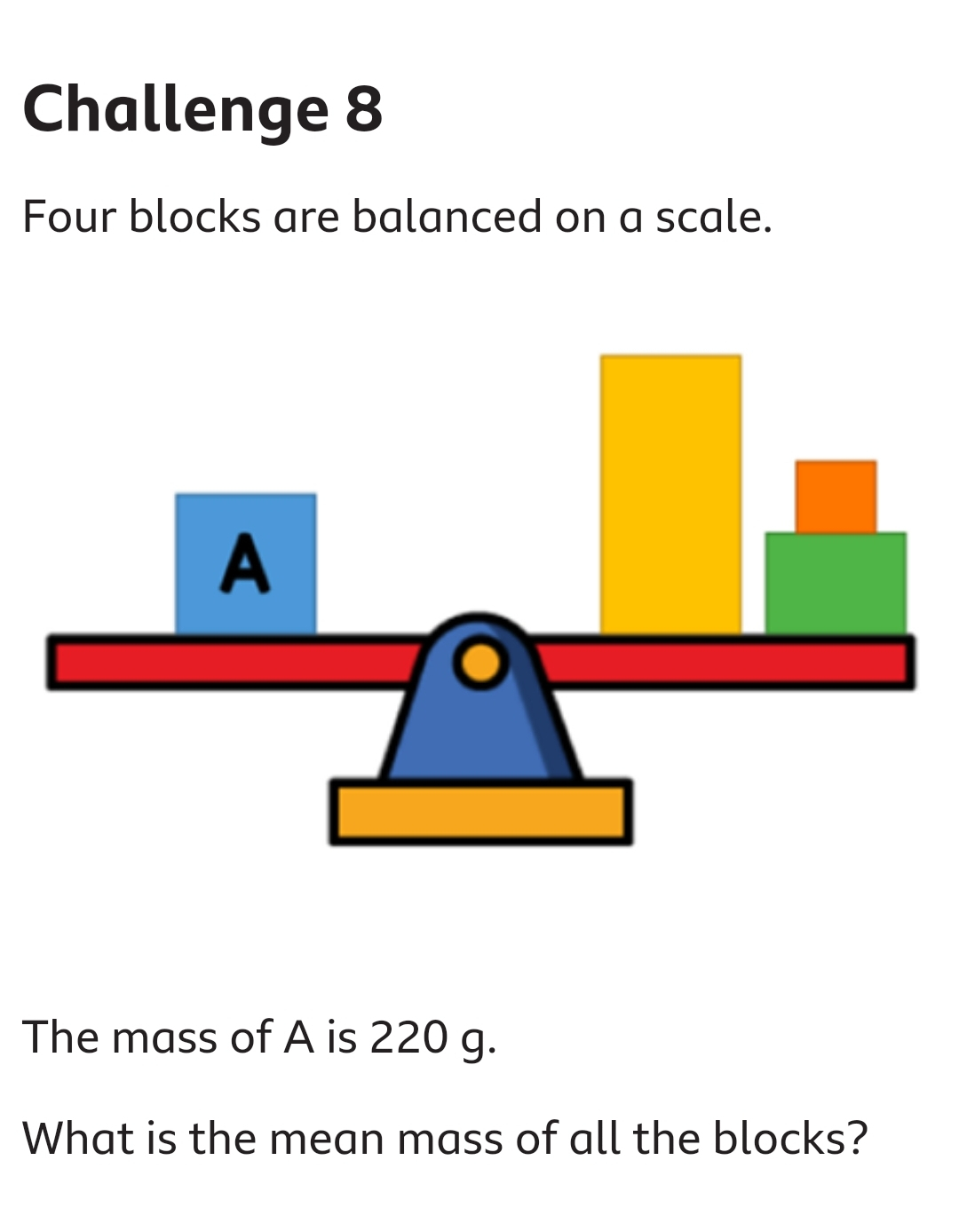# BBC bitesize maths challenge

Posted 19th Jun
Please can some one help with question in the pic.

I thought the answer was 440?110?
Jump_Out_Gang19/06/2020 10:18

110?

How? Its 220 on one side and balanced so the other side must be 220 too making it 440 in total? BBC answer is 110 but I don't understand how.
Edited by: "ran123ran" 19th Jun
ran123ran19/06/2020 10:23

How? Its 220 on one side and balanced so the other side must be 220 too …How? Its 220 on one side and balanced so the other side must be 220 too making it 440 in total? BBC answer is 110 but I don't understand how.

It asks for the mean though, so 440/4 (total mass divided by how many blocks there are) is 110
Harry_Felton19/06/2020 10:29

It asks for the mean though, so 440/4 (total mass divided by how many …It asks for the mean though, so 440/4 (total mass divided by how many blocks there are) is 110

I didn't see that little "mean" word!
Makes sense now.
Taking me back to school days when being told to read the question carefully.
I couldn’t work it out and I read the word mean
Insufficient information.

You need the density of the blocks and the relative mass centres from the balance point.
definitely 110 as stated above.Number of blocks = 4 weight = 440g when divided by 4 = 110g
i love the way they come up with maths questions. how useful is this ever going to be? in what scenario will the kid grow up to need to work out the mean weight of books balanced on a scale??
mean, median, mode

The mean means average. To find it, add together all of your values and divide by the number of addends. The median is the middle number of your data set when in order from least to greatest. The mode is the number that occurred the most often*.

mutley119/06/2020 11:29

i love the way they come up with maths questions. how useful is this ever …i love the way they come up with maths questions. how useful is this ever going to be? in what scenario will the kid grow up to need to work out the mean weight of books balanced on a scale??

Some people make a hobby out of awkward questions.
u66454119/06/2020 12:47

mean, median, modeThe mean means average. To find it, add together all of …mean, median, modeThe mean means average. To find it, add together all of your values and divide by the number of addends. The median is the middle number of your data set when in order from least to greatest. The mode is the number that occurred the most often*.*nicked from Google

don't matter. what the kid needs to learn is that when he grows up, he needs to be "above average"
u66454119/06/2020 12:47

mean, median, modeThe mean means average. To find it, add together all of …mean, median, modeThe mean means average. To find it, add together all of your values and divide by the number of addends. The median is the middle number of your data set when in order from least to greatest. The mode is the number that occurred the most often*.*nicked from Google

Technically either mean, median or mode can all be referred to as averages, although mean is by fat the most commonly used definition.